CAT  >  Trigonometry: Solved Examples

# Trigonometry: Solved Examples - Quantitative Aptitude (Quant) - CAT

Question 1: 3sinx + 4cosx + r is always greater than or equal to 0. What is the smallest value ‘r’ can to take?
A. 5
B. -5
C. 4
D. 3

Explanation-

3sinx + 4cosx ≥ -r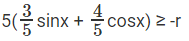3/5 = cosA ⇒ sinA = 4/5

5(sinx cosA + sinA cosx) ≥ -r

5(sin(x + A)) ≥ -r

5sin (x + A) ≥ -r

-1 ≤ sin (angle) ≤ 1

5sin (x + A) ≥ -5

rmin = 5

The question is "What is the smallest value ‘r’ can to take?"

Choice A is the correct answer.

Question 2: Sin2014x + Cos2014x = 1, x in the range of [-5π, 5π], how many values can x take?
A. 0
B. 10
C. 21
D. 11
Explanation-
We know that Sin2x + Cos2x = 1 for all values of x.

If Sin x or Cos x is equal to –1 or 1, then Sin2014x + Cos2014x will be equal to 1.

Sin x is equal to –1 or 1 when x = –4.5π or –3.5π or –2.5π or –1.5π or –0.5π or 0.5π or 1.5π or 2.5π or 3.5π or 4.5π.

Cosx is equal to –1 or 1 when x = –5π or –4π or –3π or –2π or –π or 0 or π or 2π or 3π or 4π or 5π.

For all other values of x, Sin2014 x will be strictly lesser than Sin2x.

For all other values of x, Cos2014 x will be strictly lesser than Cos2x.

We know that Sin2x + Cos2x is equal to 1. Hence, Sin2014x + Cos2014x will never be equal to 1 for all other values of x. Thus there are 21 values.

The question is "How many values the 'x' can take?"

Choice C is the correct answer.

Question 3: Consider a regular hexagon ABCDEF. There are towers placed at B and D. The angle of elevation from A to the tower at B is 30 degrees, and to the top of the tower at D is 45 degrees. What is the ratio of the heights of towers at B and D?
A. 1:√3
B. 1:2√3
C. 1:2
D. 3:4√3
Explanation-

Let the hexagon ABCDEF be of side ‘a’. Line AD = 2a. Let towers at B and D be B’B and D’D respectively.
From the given data we know that ∠B´AB = 30° and ∠D´AB = 45°. Keep in mind that the Towers B’B and D´D are not in the same plane as the hexagon.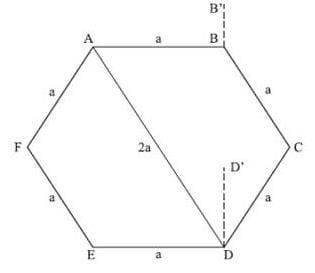In Triangle B’AB,
Tan∠B´AB = B´AB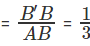B’B =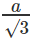In Triangle D´AD, tan ∠D´AD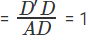D’D = 2a
Ratio of heights =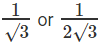The question is "What is the ratio of heights?"

Choice B is the correct answer.

Question 4: Find the maximum and minimum value of 8 cos A + 15 sin A + 15
A. 11√2+15
B. 30; 8
C. 32; -2
D. 23; 8

Explanation-
Always look out for Pythagorean triplets, we know that (8,15,17) is one
∴ The expression becomes: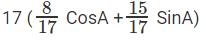Let there be a angle B for which sin B = 8/17, cos B = 15/17
⇒ 17( sin B cos A + cos B sin A) + 15
17(sin(A+B)) + 15
We know that sin(A+B)max = 1
sin(A+B)min = -1
∴ Max value = 17 * 1 +15 = 32
Min value = 17 * -1 + 15 = -2

The question is "to find the maximum and minimum value "

##### Hence, the answer is 32; -2

Choice B is the correct answer.

Question 5: : If cos A + cos2 A = 1 and a sin12 A + b sin10 A + c sin8 A + d sin6 A - 1 = 0. Find the value of a+b/c+d

A. 4
B. 3
C. 6
D.1
Explanation.

Given,
Cos A = 1 - Cos2A
Cos A = Sin2 A
Cos2A = Sin4A
1 – Sin2 A = Sin4 A
1 = Sin4 A + Sin2 A
13 = (Sin4A + Sin2A)3
1 = Sin12 A + Sin6A + 3Sin8 A + 3Sin10 A
Sin12 A + Sin6A + 3Sin8 A + 3Sin10 A – 1 = 0
On comparing,
a = 1, b = 3 , c = 3 , d = 1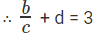Always look out for Pythagorean triplets, we know that (8,15,17) is one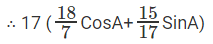The question is "Find the value of a+b/c+d"

##### Hence, the answer is 3

Choice B is the correct answer.

Question 6: In the below figure, the sheet of width W is folded along PQ such that R overlaps S Length of PQ can be written as :-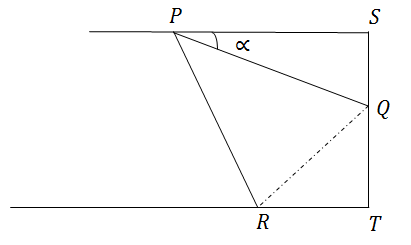A.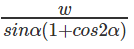B.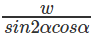C.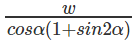D. Any two of the above
Answer: Any two of the above

Explanation-
If you are quick at observing , this question can be solved just by looking at the options as ,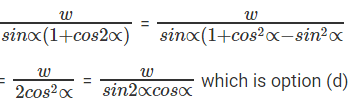Actually solving the question.
For R and S to overlap,
∆ PQR is congurent to ∆ PSQ
∴ ∠ QPR = ⁡∝ ; ∠ SQP = ∠ PQR = 90 - ⁡∝
∠ RQT = 2⁡∝ (180°-(180°-2∝))
We can draw the figure as :-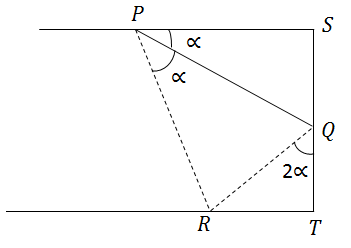∴In ∆ QRT
QT = QR cos2⁡∝
In ∆ PSQ,
SQ = PQ sin∝ = QR
∴ w = QT + SQ = PQ sin∝cos2∝ + PQ sin∝
= PQ sin∝ (1 + cos 2∝)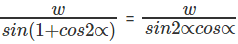(as shown earlier)

The question is "Length of PQ can be written as "

##### Hence, the answer is Any two of the above

Choice D is the correct answer.

Question 7: Ram and Shyam are 10 km apart. They both see a hot air balloon passing in the sky making an angle of 60° and 30° respectively. What is the height at which the balloon could be flying?
A.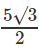B. 5√3
C. Both A and B
D. Can’t be determined
Explanation-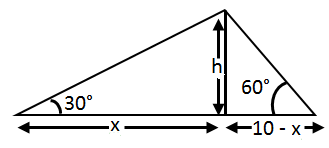tan 30° = h/x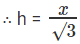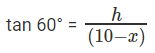h = (10 – x) √3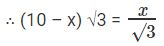30 – 3x = x ⇒ x = 30/4 = 15/2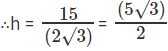Case 2 :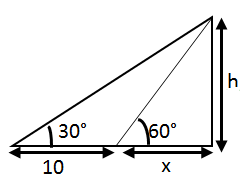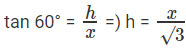Also,
tan 30° =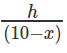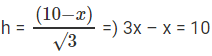x = 5
H = 5√3

The question is "What is the height at which the balloon could be flying"

##### Hence, the answer is Both A and B

Choice C is the correct answer.

Question 8: A man standing on top of a tower sees a car coming towards the tower. If it takes 20 minutes for the angle of depression to change from 30° to 60°, what is the time remaining for the car to reach the tower?
A. 20√3 minutes
B. 10 minutes
C. 10√3 minutes
D. 5 minutes

Explanation-

From the figure:-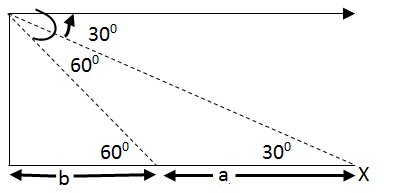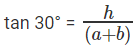tan 60° =  h/b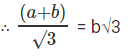a + b = 3b
2b = a
⇒ b = a/2

Now since the car takes 20 minutes to travel a distance
Time taken to travel b = a/2 distance = 20/2 = 10 minutes

The question is "What is the time remaining for the car to reach the tower?"

##### Hence, the answer is 10 minutes

Choice B is the correct answer.

Question 9: A right angled triangle has a height ‘p’, base ‘b’ and hypotenuse ‘h’. Which of the following value can h2 not take, given that p and b are positive integers?
A. 74
B. 52
C. 13
D. 23

Explanation-
We know that,
h2 = p2 + b2 Given, p and b are positive integer, so h2 will be sum of two perfect squares.
We see
a) 72 + 52 = 74
b) 62 + 42 = 52
c)  32 + 22 = 13
d) Can’t be expressed as a sum of two perfect squares

The question is "Which of the following value can h2 not take, given that p and b are positive integers? "

##### Hence, the answer is 23

Choice D is the correct answer.

Question 10: tan ∅ + sin ∅ = m, tan ∅ - sin ∅ = n, Find the value of m2- n2
A. 2√mn
B. 4√mn
C. m – n
D. 2mn

Explanation-
tan ∅ =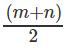Subtracting the same,
sin ∅ =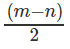Since, there are no available direct formula for relation between sin∅ ∅tan∅ but we know that

cosec2 ∅ – cos2 ∅ = 1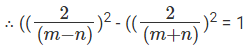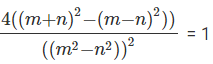⇒((m2-n2))2 = 4(4mn)
⇒ m2 - n2 = 4√mn

The question is "To find the value of m2= n2"

##### Hence, the answer is 4√mn

Choice B is the correct answer.

Question 11: A student is standing with a banner at the top of a 100 m high college building. From a point on the ground, the angle of elevation of the top of the student is 60° and from the same point, the angle of elevation of the top of the tower is 45°. Find the height of the student.
A. 35 m
B. 73.2 m
C. 50 m
D. 75 m
Explanation-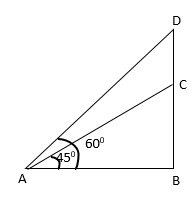Let BC be the height of the tower and DC be the height of the student.
In rt. ∆ ABC
AB = BC cot 45°
AB = 100 m ……………(i)
In rt. ∆ ABD
AB = BD cot 60°
AB = (BC + CD) cot 60°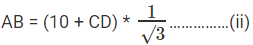Equating (i) and (ii)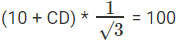(10 + CD)= 100√3
CD = 100√3 – 100
= 10(1.732 – 1) = 100 * 0.732 = 73.2 m

The question is "To find the height of the student"

##### Hence, the answer is 73.2 m

Choice B is the correct answer.

Question 12: If Cos x – Sin x = √2 Sin x, find the value of Cos x + Sin x:
A. √2 Cos x
B. √2 Cosec x
C. √2 Sec x
D. √2 Sin x Cos x

Explanation-
Cos x – Sin x = √2 Sin x
Cos x = Sin x + √2 Sin x
Cos x = Sin x + √2 Sin x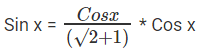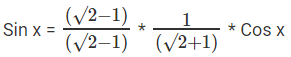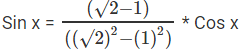Sin x = (√2 - 1) Cos x
Sin x = √2 Cos x – Cos x
Sin x + Cos x = √2 Cos x

The question is "To find the value of Cos x + Sin x"

##### Hence, the answer is √2 Cos x

Choice A is the correct answer.

Question 13: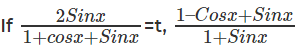can be written as:
A. 1/t
B. t
C. √t Sec x
D.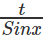Explanation.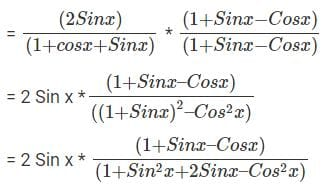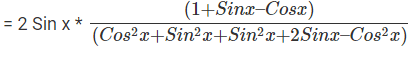(Since, 1 = Cos2x + Sin 2x)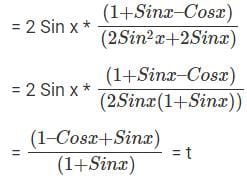The question is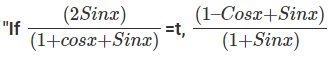can be written as"

##### Hence, the answer is t

Choice B is the correct answer.

Question 14: A tall tree AB and a building CD are standing opposite to each other. A portion of the tree breaks off and falls on top of the building making an angle of 30°. After a while it falls again to the ground in front of the building, 4 m away from foot of the tree, making an angle of 45°. The height of the building is 6 m. Find the total height of the tree in meters before it broke.
A. 27√3 + 39
B. 12√3 + 10
C. 15√3 + 21
D. Insufficient Data
Explanation-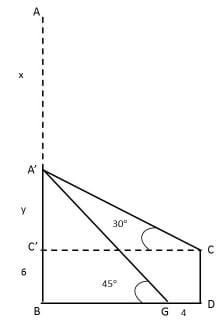Let the broken portion of tree AA’ be x. Hence A’C = A’G = x
From the figure, total height of the tree = x + y + 6
Consider triangle A’BG, tan 45° =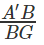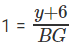Or BG = y + 6
Consider triangle A’C’C, tan 30° =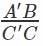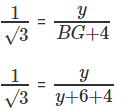y√3 = y + 10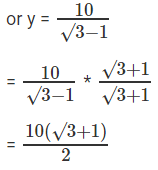Therefore y = 5(√3 + 1)
Take sin 30° to find x
sin 30° =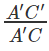1/2 = y/x
or x = 2y
Height of the tree = x + y = 6
= 2 * 5(√3 + 1) + 5(√3 + 1) + 6
= 10√3 + 10 + 5√3 + 5 + 6
= 15√3 + 21 meters

The question is "To find the total height of the tree in meters before it broke"

##### Hence, the answer is 15√3 + 21

Choice C is the correct answer.

The document Trigonometry: Solved Examples | Quantitative Aptitude (Quant) - CAT is a part of the CAT Course Quantitative Aptitude (Quant).
All you need of CAT at this link: CAT

## Quantitative Aptitude (Quant)

179 videos|158 docs|113 tests

## FAQs on Trigonometry: Solved Examples - Quantitative Aptitude (Quant) - CAT

 1. What are the basic trigonometric functions?Ans. The basic trigonometric functions are sine (sin), cosine (cos), and tangent (tan). These functions relate the angles of a right triangle to the ratios of the lengths of its sides.
 2. How can trigonometry be used to solve real-world problems?Ans. Trigonometry can be used to solve real-world problems such as finding the height of a building, the distance across a river, or the angle of elevation of an object. By applying trigonometric concepts and formulas, we can calculate unknown quantities in various scenarios.
 3. What is the unit circle and how is it used in trigonometry?Ans. The unit circle is a circle with a radius of 1 unit. It is used in trigonometry to define the values of trigonometric functions for any angle. By associating the coordinates of points on the unit circle with the values of sine and cosine, we can determine the values of these functions for all angles.
 4. How can trigonometry help in understanding waves and oscillations?Ans. Trigonometry plays a crucial role in understanding waves and oscillations. The sine and cosine functions represent waveforms, and their properties can be analyzed using trigonometry. Trigonometric concepts are used to describe wave amplitudes, frequencies, and phase shifts, making it essential in fields like physics and engineering.
 5. What are the reciprocal trigonometric functions and their applications?Ans. The reciprocal trigonometric functions are cosecant (csc), secant (sec), and cotangent (cot). These functions are the reciprocals of sine, cosine, and tangent, respectively. They find applications in various areas such as physics, engineering, and signal processing. For example, the cosecant function is used to analyze sound waves, while the secant function is used in the study of electromagnetic waves.

## Quantitative Aptitude (Quant)

179 videos|158 docs|113 testsExplore Courses for CAT exam### How to Prepare for CAT

Read our guide to prepare for CAT which is created by Toppers & the best Teachers
Signup to see your scores go up within 7 days! Learn & Practice with 1000+ FREE Notes, Videos & Tests.
10M+ students study on EduRev
Track your progress, build streaks, highlight & save important lessons and more!
Related Searches

,

,

,

,

,

,

,

,

,

,

,

,

,

,

,

,

,

,

,

,

,

;